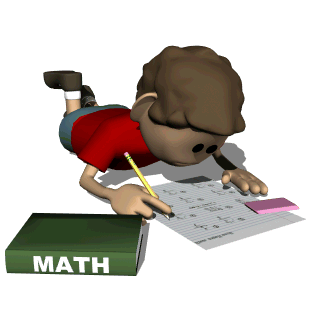•Math Facts - Multiplication Practicehttp://www.multiplication.com Fun Site - http://www.coolmath4kids.com Topics Covered word problemsplace valueroundingtimeaddition and subtraction with regroupingdata analysismultiplication and divisionpatternsgeometry and measurementfractions and decimals
Homework
• The students will have math homework Monday through Thursday. Math homework is meant as a reinforcement of the day's work. Homework will be done in the workbook or the students will be given a worksheet. Please check the homework book for daily assignments and test days.
• There will be no math homework on test days or on Fridays

Chapter Tests
• There will be a test at the conclusion of each chapter.
• Students will be given study sheets in advance.